1591460880

# PyTorch made Easy : Linear regression with PyTorch

PyTorch made Easy : Linear regression with PyTorch
In this article we are going to explain the linear regression and we will implement it from scratch with PyTorch.

#ai #artificial-intelligence #machine-learning #pytorch

## Buddha Community1594271340

## A Deep Dive into Linear Regression

Let’s begin our journey with the truth — machines never learn. What a typical machine learning algorithm does is find a mathematical equation that, when applied to a given set of training data, produces a prediction that is very close to the actual output.

Why is this not learning? Because if you change the training data or environment even slightly, the algorithm will go haywire! Not how learning works in humans. If you learned to play a video game by looking straight at the screen, you would still be a good player if the screen is slightly tilted by someone, which would not be the case in ML algorithms.

However, most of the algorithms are so complex and intimidating that it gives our mere human intelligence the feel of actual learning, effectively hiding the underlying math within. There goes a dictum that if you can implement the algorithm, you know the algorithm. This saying is lost in the dense jungle of libraries and inbuilt modules which programming languages provide, reducing us to regular programmers calling an API and strengthening further this notion of a black box. Our quest will be to unravel the mysteries of this so-called ‘black box’ which magically produces accurate predictions, detects objects, diagnoses diseases and claims to surpass human intelligence one day.

We will start with one of the not-so-complex and easy to visualize algorithm in the ML paradigm — Linear Regression. The article is divided into the following sections:

1. Need for Linear Regression

2. Visualizing Linear Regression

3. Deriving the formula for weight matrix W

4. Using the formula and performing linear regression on a real world data set

Note: Knowledge on Linear Algebra, a little bit of Calculus and Matrices are a prerequisite to understanding this article

Also, a basic understanding of python, NumPy, and Matplotlib are a must.

## 1) Need for Linear regression

Regression means predicting a real valued number from a given set of input variables. Eg. Predicting temperature based on month of the year, humidity, altitude above sea level, etc. Linear Regression would therefore mean predicting a real valued number that follows a linear trend. Linear regression is the first line of attack to discover correlations in our data.

Now, the first thing that comes to our mind when we hear the word linear is, a line.

Yes! In linear regression, we try to fit a line that best generalizes all the data points in the data set. By generalizing, we mean we try to fit a line that passes very close to all the data points.

But how do we ensure that this happens? To understand this, let’s visualize a 1-D Linear Regression. This is also called as Simple Linear Regression

#calculus #machine-learning #linear-regression-math #linear-regression #linear-regression-python #python1598352300

## Regression: Linear Regression

Machine learning algorithms are not your regular algorithms that we may be used to because they are often described by a combination of some complex statistics and mathematics. Since it is very important to understand the background of any algorithm you want to implement, this could pose a challenge to people with a non-mathematical background as the maths can sap your motivation by slowing you down.In this article, we would be discussing linear and logistic regression and some regression techniques assuming we all have heard or even learnt about the Linear model in Mathematics class at high school. Hopefully, at the end of the article, the concept would be clearer.

**Regression Analysis **is a statistical process for estimating the relationships between the dependent variables (say Y) and one or more independent variables or predictors (X). It explains the changes in the dependent variables with respect to changes in select predictors. Some major uses for regression analysis are in determining the strength of predictors, forecasting an effect, and trend forecasting. It finds the significant relationship between variables and the impact of predictors on dependent variables. In regression, we fit a curve/line (regression/best fit line) to the data points, such that the differences between the distances of data points from the curve/line are minimized.

#regression #machine-learning #beginner #logistic-regression #linear-regression #deep learning1592023980

## 5 Regression algorithms: Explanation & Implementation in Python

Take your current understanding and skills on machine learning algorithms to the next level with this article. What is regression analysis in simple words? How is it applied in practice for real-world problems? And what is the possible snippet of codes in Python you can use for implementation regression algorithms for various objectives? Let’s forget about boring learning stuff and talk about science and the way it works.

#linear-regression-python #linear-regression #multivariate-regression #regression #python-programming1596682860

## Linear Regression with PyTorch

Your first step towards deep learningIn my previous posts we have gone through

Here we will try to solve the classic linear regression problem using pytorch tensors.

1 What is Linear regression ?

y = Ax + B.

A = slope of curve

B = bias (point that intersect y-axis)

y=target variable

x=feature variable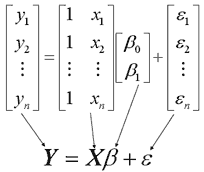Linear regression matrix (Source : https://online.stat.psu.edu/stat462/node/132/)

1.1 Linear regression example 1:

We’ll create a model that predicts crop yields for apples and oranges (target variables) by looking at the average temperature, rainfall and humidity (input variables or features) in a region. Here’s the training data: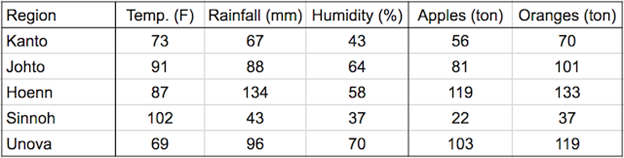yield_apple = w11 * temp + w12 * rainfall + w13 * humidity + b1

yield_orange = w21 * temp + w22 * rainfall + w23 * humidity + b2

Learning rate of linear regression is to find out these w,b values to make accurate predictions on unseen data. This optimization technique for linear regression is gradient descent which slightly adjusts weights many times to make better predictions.Below is the matrix representation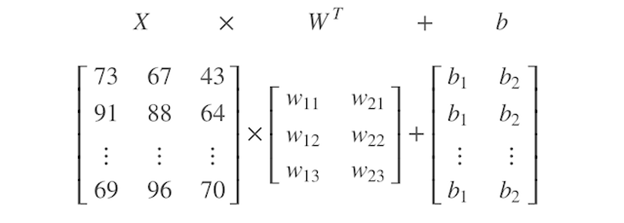#python #pytorch #linear-regression #neural-networks #deep-learning1597277100

## Linear Regression in PyTorch

### A brief introduction to Linear Regression in PyTorch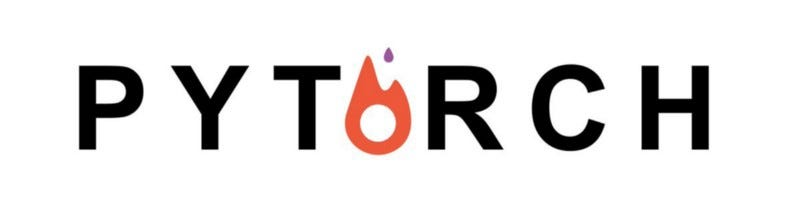For all those amateur Machine Learning and Deep Learning enthusiasts out there, Linear Regression is just the right way to kick start your journey. If you are new to Machine Learning with some background in PyTorch, then buckle up because you have just ended up at the right spot. In case you are not, don’t worry, checkout my previous article to pick up some fundamentals of PyTorch and then you are all good to go.

## So what is Linear Regression ?

Linear Regression is a linear model that predicts the output, given a set of input variables, assuming that there is a linear relationship between the input variables and the single output variable.

For instance let’s just consider the simple linear equation y = w*x + b. Here y is the output and x is the input variable. “w” and “b” forms the slope and y intercept of the equation respectively. So we will refer to “w” and “b” as the parameters of the equation because once you get to know these values, you can easily predict the output for a given value of x.

Now let’s slightly change the scenario. Assume that you are given the values of x and y (we will call them the training set ) and you are asked to find out the new value of y corresponding to a new variable x. Obviously, simple linear algebra would do the trick and easily give you the right parameters . Once you plug them into the equation you can find out the new value of y, corresponding to x, in no time.

So what’s the big deal, these are things that we have covered in our high school. But here is what you should consider:

1. Real world datasets may have noise in them i.e. there may be some values of x and y, in your training set, that would not go hand in hand with some others values of x and y in the same set, obviously putting an end to your attempt to find out the right values of the parameters using the traditional approach.
2. Also in real world datasets there may be more than one variable determining an output variable thus it becomes a hefty task to find out the parameters when several input variables are involved.

This is where Machine learning steps in. I will try to give you an overview of what is happening behind the scenes of the infamous Linear regression method. Initially our Machine know nothing more than an average child. It will take some random values of “w” and “b”, which from now on we will refer to as the weight and the bias, and plug those into the equation y = w*x + b. Now it will take some values of x in the training set and find out the corresponding values of y using the parameters that it had assumed earlier. It will then compare the predicted values of y say yhat, with the actual values of y by calculating something known as the loss function (cost function).

The loss function essentially denotes the error in our prediction. A greater value of the loss function denotes a greater error. Here we will be using Mean Square Error (MSE) as our loss function and it is given by the formula :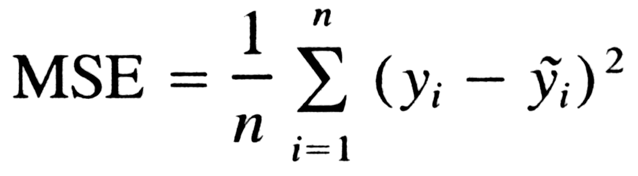Once the loss is calculated the we perform an optimization method like gradient descent on the loss function. Stuck with gradient descent, don’t worry, for the time sake all you have to know is that gradient descent is simply a method performed on the loss function to find the values of “w” and “b” that minimizes the loss function. It involves the use of learning rate which can be tweaked for better results. Smaller the loss more accurate is our prediction.

#deep-learning #pytorch #linear-regression #machine-learning #deep learning